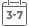# Financial Statistics and Mathematical Finance: Methods, Models and Applications

• Publish Date: 2012-08-13
• Binding: Hardcover
• Author: Ansgar Steland
• Sale
• Regular price \$134.30Mathematical finance has grown into a huge area of research which requires a lot of care and a large number of sophisticated mathematical tools. Mathematically rigorous and yet accessible to advanced level practitioners and mathematicians alike, it considers various aspects of the application of statistical methods in finance and illustrates some of the many ways that statistical tools are used in financial applications.

Financial Statistics and Mathematical Finance:

• Provides an introduction to the basics of financial statistics and mathematical finance.
• Explains the use and importance of statistical methods in econometrics and financial engineering.
• Illustrates the importance of derivatives and calculus to aid understanding in methods and results.
• Looks at advanced topics such as martingale theory, stochastic processes and stochastic integration.
• Features examples throughout to illustrate applications in mathematical and statistical finance.
• Is supported by an accompanying website featuring R code and data sets.

Financial Statistics and Mathematical Finance introduces the financial methodology and the relevant mathematical tools in a style that is both mathematically rigorous and yet accessible to advanced level practitioners and mathematicians alike, both graduate students and researchers in statistics, finance, econometrics and business administration will benefit from this book.

MORE FROM THIS COLLECTION